# Boundary Fill Algorithm

Prerequisite : Flood fill algorithm, Scan-line polygon filling

Introduction : Boundary Fill Algorithm starts at a pixel inside the polygon to be filled and paints the interior proceeding outwards towards the boundary. This algorithm works only if the color with which the region has to be filled and the color of the boundary of the region are different. If the boundary is of one single color, this approach proceeds outwards pixel by pixel until it hits the boundary of the region.

Attention reader! Don’t stop learning now. Get hold of all the important DSA concepts with the DSA Self Paced Course at a student-friendly price and become industry ready.  To complete your preparation from learning a language to DS Algo and many more,  please refer Complete Interview Preparation Course.

In case you wish to attend live classes with experts, please refer DSA Live Classes for Working Professionals and Competitive Programming Live for Students.

Boundary Fill Algorithm is recursive in nature. It takes an interior point(x, y), a fill color, and a boundary color as the input. The algorithm starts by checking the color of (x, y). If it’s color is not equal to the fill color and the boundary color, then it is painted with the fill color and the function is called for all the neighbours of (x, y). If a point is found to be of fill color or of boundary color, the function does not call its neighbours and returns. This process continues until all points up to the boundary color for the region have been tested.

The boundary fill algorithm can be implemented by 4-connected pixels or 8-connected pixels.

4-connected pixels : After painting a pixel, the function is called for four neighboring points. These are the pixel positions that are right, left, above and below the current pixel. Areas filled by this method are called 4-connected. Below given is the algorithm :
Algorithm :

```void boundaryFill4(int x, int y, int fill_color,int boundary_color)
{
if(getpixel(x, y) != boundary_color &&
getpixel(x, y) != fill_color)
{
putpixel(x, y, fill_color);
boundaryFill4(x + 1, y, fill_color, boundary_color);
boundaryFill4(x, y + 1, fill_color, boundary_color);
boundaryFill4(x - 1, y, fill_color, boundary_color);
boundaryFill4(x, y - 1, fill_color, boundary_color);
}
}
```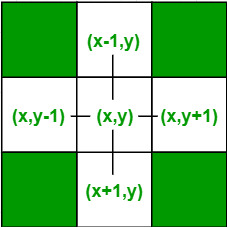Below is the implementation of above algorithm :

 `// C Implementation for Boundary Filling Algorithm``#include `` ` `// Function for 4 connected Pixels``void` `boundaryFill4(``int` `x, ``int` `y, ``int` `fill_color,``int` `boundary_color)``{``    ``if``(getpixel(x, y) != boundary_color &&``       ``getpixel(x, y) != fill_color)``    ``{``        ``putpixel(x, y, fill_color);``        ``boundaryFill4(x + 1, y, fill_color, boundary_color);``        ``boundaryFill4(x, y + 1, fill_color, boundary_color);``        ``boundaryFill4(x - 1, y, fill_color, boundary_color);``        ``boundaryFill4(x, y - 1, fill_color, boundary_color);``    ``}``}`` ` `//driver code``int` `main()``{``    ``// gm is Graphics mode which is``    ``// a computer display mode that``    ``// generates image using pixels.``    ``// DETECT is a macro defined in``    ``// "graphics.h" header file``    ``int` `gd = DETECT, gm;`` ` `    ``// initgraph initializes the``    ``// graphics system by loading a``    ``// graphics driver from disk``    ``initgraph(&gd, &gm, ``""``);`` ` `    ``int` `x = 250, y = 200, radius = 50;`` ` `    ``// circle function``    ``circle(x, y, radius);`` ` `    ``// Function calling``    ``boundaryFill4(x, y, 6, 15);`` ` `    ``delay(10000);`` ` `    ``getch();`` ` `    ``// closegraph function closes the``    ``// graphics mode and deallocates``    ``// all memory allocated by``    ``// graphics system .``    ``closegraph();`` ` `    ``return` `0;``}`

Output :

```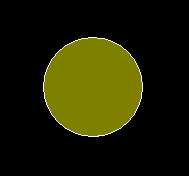```

8-connected pixels : More complex figures are filled using this approach. The pixels to be tested are the 8 neighboring pixels, the pixel on the right, left, above, below and the 4 diagonal pixels. Areas filled by this method are called 8-connected. Below given is the algorithm :
Algorithm :

```void boundaryFill8(int x, int y, int fill_color,int boundary_color)
{
if(getpixel(x, y) != boundary_color &&
getpixel(x, y) != fill_color)
{
putpixel(x, y, fill_color);
boundaryFill8(x + 1, y, fill_color, boundary_color);
boundaryFill8(x, y + 1, fill_color, boundary_color);
boundaryFill8(x - 1, y, fill_color, boundary_color);
boundaryFill8(x, y - 1, fill_color, boundary_color);
boundaryFill8(x - 1, y - 1, fill_color, boundary_color);
boundaryFill8(x - 1, y + 1, fill_color, boundary_color);
boundaryFill8(x + 1, y - 1, fill_color, boundary_color);
boundaryFill8(x + 1, y + 1, fill_color, boundary_color);
}
}
```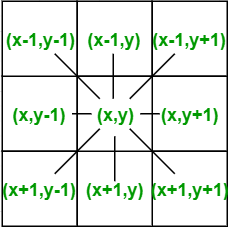Below is the implementation of above algorithm :

 `// C Implementation for Boundary Filling Algorithm``#include `` ` `// Function for 8 connected Pixels``void` `boundaryFill8(``int` `x, ``int` `y, ``int` `fill_color,``int` `boundary_color)``{``    ``if``(getpixel(x, y) != boundary_color &&``       ``getpixel(x, y) != fill_color)``    ``{``        ``putpixel(x, y, fill_color);``        ``boundaryFill8(x + 1, y, fill_color, boundary_color);``        ``boundaryFill8(x, y + 1, fill_color, boundary_color);``        ``boundaryFill8(x - 1, y, fill_color, boundary_color);``        ``boundaryFill8(x, y - 1, fill_color, boundary_color);``        ``boundaryFill8(x - 1, y - 1, fill_color, boundary_color);``        ``boundaryFill8(x - 1, y + 1, fill_color, boundary_color);``        ``boundaryFill8(x + 1, y - 1, fill_color, boundary_color);``        ``boundaryFill8(x + 1, y + 1, fill_color, boundary_color);``    ``}``}`` ` `//driver code``int` `main()``{``    ``// gm is Graphics mode which is``    ``// a computer display mode that``    ``// generates image using pixels.``    ``// DETECT is a macro defined in``    ``// "graphics.h" header file``    ``int` `gd = DETECT, gm;`` ` `    ``// initgraph initializes the``    ``// graphics system by loading a``    ``// graphics driver from disk``    ``initgraph(&gd, &gm, ``""``);`` ` `    ``// Rectangle function``    ``rectangle(50, 50, 100, 100);`` ` `    ``// Function calling``    ``boundaryFill8(55, 55, 4, 15);`` ` `    ``delay(10000);`` ` `    ``getch();`` ` `    ``// closegraph function closes the``    ``// graphics mode and deallocates``    ``// all memory allocated by``    ``// graphics system .``    ``closegraph();`` ` `    ``return` `0;``}`

Output :

```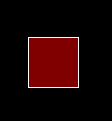```

4-connected pixels Vs 8-connected pixels :
Let us take a figure with the boundary color as GREEN and the fill color as RED. The 4-connected method fails to fill this figure completely. This figure will be efficiently filled using the 8-connected technique.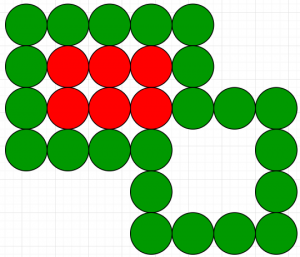Flood fill Vs Boundary fill :
Though both Flood fill and Boundary fill algorithms color a given figure with a chosen color, they differ in one aspect. In Flood fill, all the connected pixels of a selected color get replaced by a fill color. On the other hand, in Boundary fill, the program stops when a given color boundary is found.

My Personal Notes arrow_drop_up